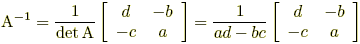# General Mathematics, Linear algebra, and Calculus.

In Machine Learning we use few mathematical terms that are important and frequently used, with this article I tried to list down those important keywords. When we walk on ML, we need a clear visualization of these terms so that we can understand what we want to achieve by using the algorithms. Machine learning is a like a toy if we read it without the deep understanding of Derivative, vectors, and probability. Derivative help in understanding the minimization of the cost function, Vectors help in understanding the overall movement and probability helps in understanding the occurrence of a conditional event.

#### Vectors

There are few quantities in the universe which we cannot define only with magnitude. We need direction as well like if we say we are applying force we need to know the direction as well. There are many terms related to vector we discuss one by one.

#### Codirected vectors

Two collinear vectors a and b are called codirected vector if their directions are the same: a↑↑b

#### Colinear Vector:

Vector parallel to one line or lying on one line are called Colinear vector.

#### Zero vector

It is a vector whose start and end points coincide.

#### Matrix

In mathematics, a matrix is a rectangular array of numbers arranged in rows and columns. In python, Numpy holds the power to sketch n-dimensional array.The matrix can hold attributes/features that are placed in columns. So a single row represents an event with a set of features.

```import numpy as np
mat=np.arange(36).reshape(6,6)```
```array([[ 0,  1,  2,  3,  4,  5],
[ 6,  7,  8,  9, 10, 11],
[12, 13, 14, 15, 16, 17],
[18, 19, 20, 21, 22, 23],
[24, 25, 26, 27, 28, 29],
[30, 31, 32, 33, 34, 35]])```

```import numpy as np
mat1=np.ones([6,6])
mat2=np.ones([6,6])+2```
```array([[ 4.,  4.,  4.,  4.,  4.,  4.],
[ 4.,  4.,  4.,  4.,  4.,  4.],
[ 4.,  4.,  4.,  4.,  4.,  4.],
[ 4.,  4.,  4.,  4.,  4.,  4.],
[ 4.,  4.,  4.,  4.,  4.,  4.],
[ 4.,  4.,  4.,  4.,  4.,  4.]])```

#### Dot and Cross product

```import numpy as np
mat1=np.ones([6,6])
mat2=np.ones([6,6])+2
#Dot product
np.dot(mat2,mat1)
#Cross product
mat2*mat1```

#### Linear independent vector

```import numpy as np

matrix = np.array(
[
[0, 1 ,0 ,0],
[0, 0, 1, 0],
[0, 1, 1, 0],
[1, 0, 0, 1]
])

lambdas, V =  np.linalg.eig(matrix.T)
# The linearly dependent row vectors
print(matrix[lambdas == 0,:])```

#### Rank of a Matrix

One of the most important concepts in linear algebra is the rank of a matrix. The rank of a matrix is the number of linearly independent column vectors or row vectors. The number of independent columns
vectors would always be equal to the number of independent row vectors for a matrix.

```from numpy import  rank
A = matrix([[1,3,7],[2,8,3],[7,8,1]])
print (np.linalg.matrix_rank(A))```

#### Identity Matrix

The identity array is a square array with ones on the main diagonal.

```np.identity(4)

array([[ 1.,  0.,  0.,  0.],
[ 0.,  1.,  0.,  0.],
[ 0.,  0.,  1.,  0.],
[ 0.,  0.,  0.,  1.]])```

#### Determinant

A determinant of a square matrix A is a number and is denoted by det(A). The determinant is the absolute value of the determinant of a matrix determines the volume enclosed by the
row vectors acting as edges.

```#Determinant
mat=[[1,2,3],[4,3,4],[2,2,3]]
print(np.linalg.det(mat))```

To find the adjoint of a matrix, first, find the cofactor matrix of the given matrix. Then find the transpose of the cofactor matrix.

cofactor—->Transpose

#### The inverse of a Matrix

When the cofactor divide by the determinant.#### EigenValue vs EigenVector

This is one of the most important and core topic of Machine learning. Eigen is used from Principal component analysis to google page ranking algorithm.

A·v=λ·v

In this equation A is an n-by-n matrix, v is a non-zero n-by-1 vector and λ is a scalar (which may be either real or complex).  Any value of λ for which this equation has a solution is known as an eigenvalue of the matrix A.  It is sometimes also called the characteristic value.  The vector, v, which corresponds to this value is called an eigenvector.  The eigenvalue problem can be rewritten as

A·v-λ·v=0